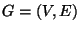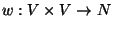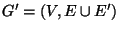Next: MINIMUM STRONG CONNECTIVITY AUGMENTATION Up: Cuts and Connectivity Previous: MINIMUM K-EDGE CONNECTED SUBGRAPH   Index

### MINIMUM BICONNECTIVITY AUGMENTATION

• INSTANCE: Graphand a symmetric weight function.

• SOLUTION: A connectivity augmenting set E' for G, i.e., a set E' of unordered pairs of vertices from V such thatis biconnected.

• MEASURE: The weight of the augmenting set, i.e.,.

• Good News: Approximable within 2  and .

• Comment: The same bound is valid also when G' must be bridge connected (edge connected) . Minimum k-Connectivity Augmentation, the problem in which G' has to be k-connected (vertex or edge connected), is also approximable within 2 . If the weight function satisfies the triangle inequality, the problem is approximable within 3/2 . Variation in which G is planar and the augmentation must be planar is approximable within 5/3 in the unweighted case (where w(u,v)=1) . Ifthe problem is the same as weighted MINIMUM K-VERTEX CONNECTED SUBGRAPH.

• Garey and Johnson: ND18

Viggo Kann
2000-03-20